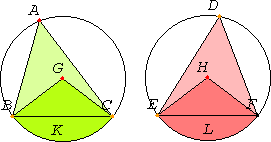Proposition 26

In equal circles equal angles stand on equal circumferences whether they stand at the centers or at the circumferences.

Let ABC and DEF be equal circles, and in them let there be equal angles, namely at the centers the angles BGC and EHF, and at the circumferences the angles BAC and EDF.

I say that the circumference BKC equals the circumference ELF.Join BC and EF.

Now, since the circles ABC and DEF are equal, the radii are equal.

I.4

Thus the two straight lines BG and GC equal the two straight lines EH and HF, and the angle at G equals the angle at H, therefore the base BC equals the base EF.

III.Def.11

And, since the angle at A equals the angle at D, the segment BAC is similar to the segment EDF, and they are upon equal straight lines.

III.24

But similar segments of circles on equal straight lines equal one another, therefore the segment BAC equals EDF. But the whole circle ABC also equals the whole circle DEF, therefore the remaining circumference BKC equals the circumference ELF.

Therefore in equal circles equal angles stand on equal circumferences whether they stand at the centers or at the circumferences.

Q.E.D.

Guide

This proposition is used in III.28, IV.11, IV,15, and XIII.10.